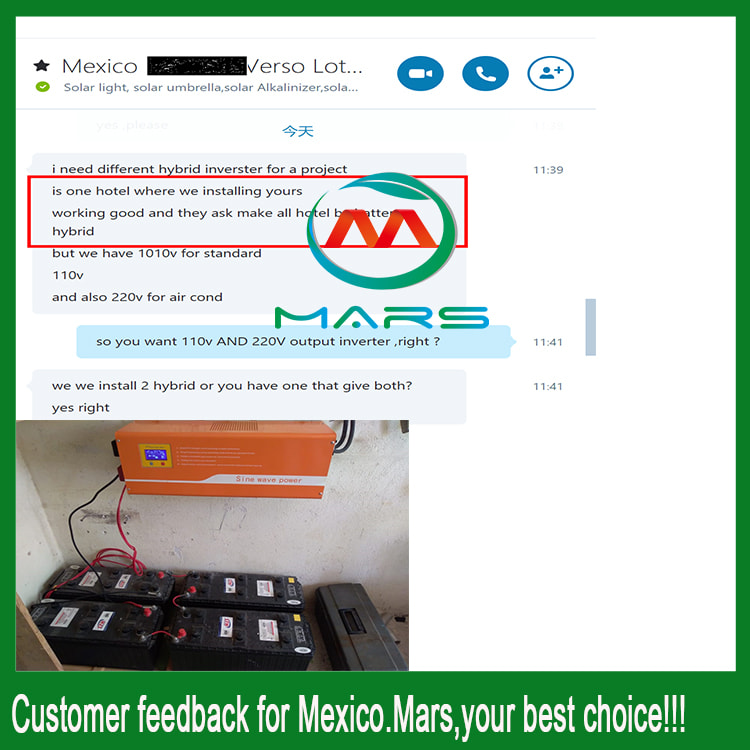Home / News / News /

# Interpretation of the terminology of the solar inverter system

0

Active power in solar inverter system: In an AC circuit, the part of the power (such as thermal energy, light energy, or mechanical energy) that is irreversibly converted to power consumed on the resistive element is called active power.Reactive power in solar inverter system: In a circuit, an inductive component establishes a magnetic field. The power that the capacitive component creates by the electric field is called no power. This power is converted with the continuous power of the power supply with the cycle of the alternating current, and does not consume energy.

Apparent power in solar inverter system: The total power that AC power can provide is called apparent power. In terms of value, it is the product of voltage and current. Unit VA, apparent power is the capacity of AC power.Resistive load:That is, when the load current load voltage has no phase difference compared with the power supply, the load is resistive (for example, the load is light, an fan, etc.)In a nutshell, a purely resistive load that works only with resistive components is called a resistive load.

Inductive load:Usually, the load with the inductance parameter of the load, that is, the load that meets the characteristics of the phase difference of the load current lags the load voltage with the power supply is inductive (such as the load is the motor; the transformer;). In layman's terms, high-power electrical products such as electric motors, compressors, relays, fluorescent lamps, etc., which are fabricated using the principle of electromagnetic induction.This type of product requires a much larger starting current (about 3-7 times) than the current required to maintain normal operation. For example, a refrigerator that consumes about 150 watts of electricity during normal operation can have a starting power of more than 1000 watts,then need to use more than 1000watts solar inverter system.

In addition, since the inductive load is connected to the pure sine wave power inverter or disconnected from the pure sine wave power inverter, a potential voltage is generated, and the peak value of the voltage is far greater than the voltage value that the AC pure sine wave power inverter can withstand, which easily causes instantaneous overload of the pure sine wave power inverter. Affect the life of the pure sine wave power inverter. Therefore, such appliances have higher requirements for power supply waveforms,a true sine wave inverter low frequency is recommended.

Latest posts
News
Hot Product